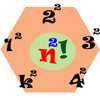# Resources tagged with: Factorials

Filter by: Content type:
Age range:
Challenge level:

### There are 11 results

Broad Topics > Numbers and the Number System > Factorials### Factorial Fun

##### Age 16 to 18 Challenge Level:

How many divisors does factorial n (n!) have?### Squaresearch

##### Age 14 to 16 Challenge Level:

Consider numbers of the form un = 1! + 2! + 3! +...+n!. How many such numbers are perfect squares?### N000ughty Thoughts

##### Age 14 to 16 Challenge Level:

How many noughts are at the end of these giant numbers?### Fac-finding

##### Age 14 to 16 Challenge Level:

Lyndon chose this as one of his favourite problems. It is accessible but needs some careful analysis of what is included and what is not. A systematic approach is really helpful.### Factorial

##### Age 14 to 16 Challenge Level:

How many zeros are there at the end of the number which is the product of first hundred positive integers?### Binomial Coefficients

##### Age 14 to 18

An introduction to the binomial coefficient, and exploration of some of the formulae it satisfies.### The Harmonic Triangle and Pascal's Triangle

##### Age 16 to 18

The harmonic triangle is built from fractions with unit numerators using a rule very similar to Pascal's triangle.### Growing

##### Age 16 to 18 Challenge Level:

Which is larger: (a) 1.000001^{1000000} or 2? (b) 100^{300} or 300! (i.e.factorial 300)### Seriesly

##### Age 16 to 18 Short Challenge Level:

Prove that k.k! = (k+1)! - k! and sum the series 1.1! + 2.2! + 3.3! +...+n.n!### Seriesly

##### Age 16 to 18 Challenge Level:

Prove that k.k! = (k+1)! - k! and sum the series 1.1! + 2.2! + 3.3! +...+n.n!### How Does Your Function Grow?

##### Age 16 to 18 Challenge Level:

Compares the size of functions f(n) for large values of n.